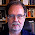## Saturday, April 25, 2009

### When There Is Not Enough Data

I have a dataset where the target (continuous variable) variable that has to be estimated. However, in the given dataset, values for target are preset only for 2% while rest of 98% do not have values. The 98% are empty values. I need to score a dataset and give values for the target for all 2500 records. Can I use the 2% and replicate it several times and use that dataset to build a model? The ASE is too high if I use the 2% data alone. Any suggestions how to handle it, please?
Thanks,
Sneha

Sneha,

The short answer to your question is "Yes, you can replicate the 2% and use it to build a model." BUT DO NOT DO THIS! Just because a tool or technique is possible to implement does not mean that it is a good idea. Replicating observations "confuses" models, often by making the model appear overconfident in its results.

Given the way that ASE (average squared error) is calculated, I don't think that replicating data is going to change the value. We can imagine adding a weight or frequency on each observation instead of replicating them. When the weights are all the same, they cancel out in the ASE formula.

What does change is confidence in the model. So, if you are doing a regression and looking at the regression coefficients, each has a confidence interval. By replicating the data, the resulting model would have smaller confidence intervals. However, these are false, because the replicated data has no more information than the original data.

The problem that you are facing is that the modeling technique you are using is simply not powerful enough to represent the 50 observations that you have. Perhaps a different modeling technique would work better, although you are working with a small amount of data. For instance, perhaps some sort of nearest neighbor approach would work well and be easy to implement.

You do not say why you are using ASE (average squared error) as the preferred measure of model fitness. I can speculate that you are trying to predict a number, perhaps using a regression. One challenge is that the numbers being predicted often fall into a particular range (such as positive numbers for dollar values or ranging between 0 and 1 for a percentage). However, regressions produce numbers that run the gamut of values. In this case, transforming the target variable can sometimes improve results.

In our class on data mining (Data Mining Techniques: Theory and Practice), Michael and I introduce the idea of oversamping rare data using weights in order to get a balanced model set. For instance, if you were predicting whether someone was in the 2% group, you might give each of them a weight of 49 and all the unknowns a weight of 1. The result would be a balanced model set. However, we strongly advise that the maximum weight be 1. So, the weights would be 1/49 for the common cases and 1 for the rare ones. For regressions, this is important because it prevents any coefficients from having too-narrow confidence intervals.

#### 1 comment:

1.The first question that needs to be answered is "why is the target missing in 98% of the cases"? What in the physical process of generating the caused the data to be missing?
Without knowing the answer to that question, there is no way to have a meaningful solution to the prediction problem.

Techniques and algorithms are great, but sometimes you have to actually think about the data :-)

Your comment will appear when it has been reviewed by the moderators.